# 嵌入式系统导论 Solutions for Priority Inversion in Real-time Scheduling

Real-time systems are collections of tasks where in addition to any ordering constraints imposed by precedences between the tasks, there are also timing constraints, requiring a scheduling strategy. Classical real-time scheduling（实时调度） for Periodic Tasks contains Periodic Rate Monotonic (RM) as well as Earliest Deadline First (EDF). What’s more, tasks share resources and use mutual exclusion to guard access to those resources, resulting in Scheduling Anomalies（调度异常）.
本文将讲述 Resources Sharingreal-time scheduling 带来的 Priority Inversion（优先级反转）异常，并将重点介绍对应的解决办法：Priority Inheritance Protocol (PIP)、Priority Ceiling Protocol (PCP)、Stack Resource Policy (SRP)。

### Priority Inversion

• Resource Sharing & Task Blocked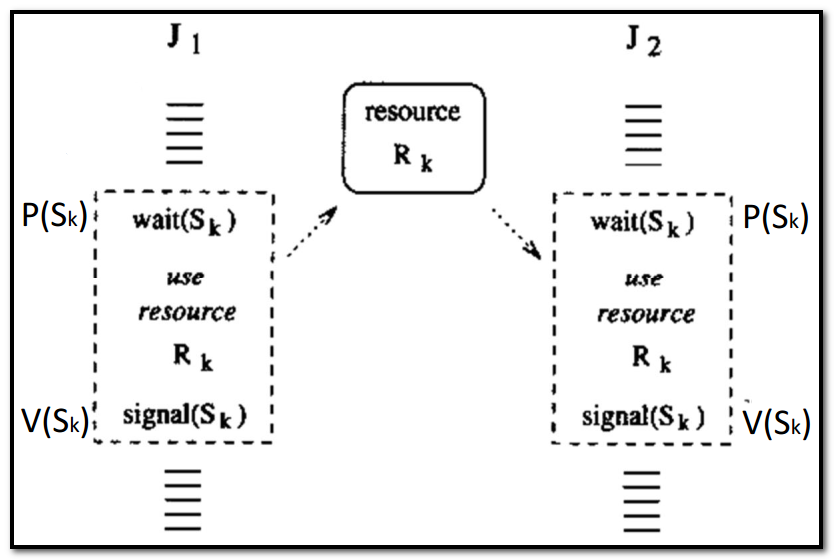• Threads (or Processes): $J_1$, $J_2$.
• Common resources $R_k$ likes: data structures, variables, main memory area, file, set of registers, I/O unit, …
• Many shared resources do not allow simultaneous accesses but require mutual exclusion (exclusive resources). A piece of code executed under mutual exclusion ($P(S_k) \leftrightarrow V(S_k)$) constraints is called a critical section.
• Each exclusive resource $R_k$ must be protected by a different semaphore $S_k$ and each critical section operating on a resource must begin with a wait($S_k$) primitive and end with a signal($S_k$) primitive（原子操作）.
• Tasks waiting for an exclusive resource is said to be blocked on that resource. Otherwise, it proceeds by entering the critical section and holds the resource. When a task leaves a critical section, the associated resource becomes free.
• All tasks blocked on the same resource are kept in a queue associated with the semaphore.
• When a running task executes a wait on a locked semaphore, it enters a waiting state, until another tasks executes a signal primitive that unlocks the semaphore.
• Priority Inversion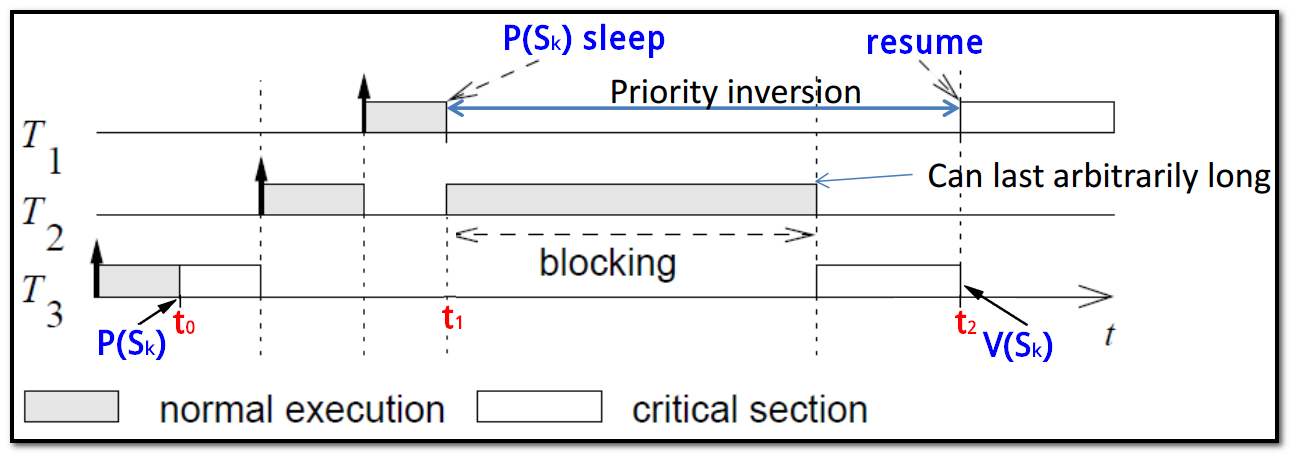• priority of $T_1$ > priority of $T_2$ > priority of $T_3$
• $T_3$ requests exclusive access first (at $t_0$), $T_1$ has to wait until $T_3$ releases the resource (at $t_2$)
• $T_2$ preempts $T_3$ (at $t_1$): $T_2$ can prevent $T_3$ from releasing the resource.
• If $T_1$ is blocked only by $T_3$, blocking is bounded by the length of the critical section, but blocked by >2 tasks ($T_3$, $T_2$, …), blocking can exceed the length of any critical section.

Priority Inversion is a scheduling anomaly where a low priority task was holding a lock and blocking a high-priority task, while medium priority tasks, unrelated lower-priority tasks, are executing.

• Solving Priority Inversion: Resource Access Protocols
• Basic idea: Modify the priority of those tasks that cause blocking.
• When a task $T_i$ blocks one or more higher priority tasks, it temporarily assumes a higher priority.
• Methods
• Priority Inheritance Protocol (PIP), for static priorities
• Priority Ceiling Protocol (PCP), for static priorities
• Stack Resource Policy (SRP), for static and dynamic priorities

static priorities（静态优先级）: 静态优先级是在创建进程时确定的，且在进程的整个运行期间保持不变，内核不会主动修改它。
dynamic priorities（动态优先级）: 在创建进程时所赋予的优先级，是可以随进程的推进或随其等待时间的增加而改变的，调度程序增大或减少进程静态优先级来奖励 IO 消耗型进程或惩罚 CPU 消耗进程以便获得更好的调度性能；调整后的优先级为动态优先级。
real-time priorities（实时优先级）: 实时优先级只对实时进程有效。

### Priority Inheritance Protocol (PIP)

• Avoid Priority Inversion in previous example uisng PIP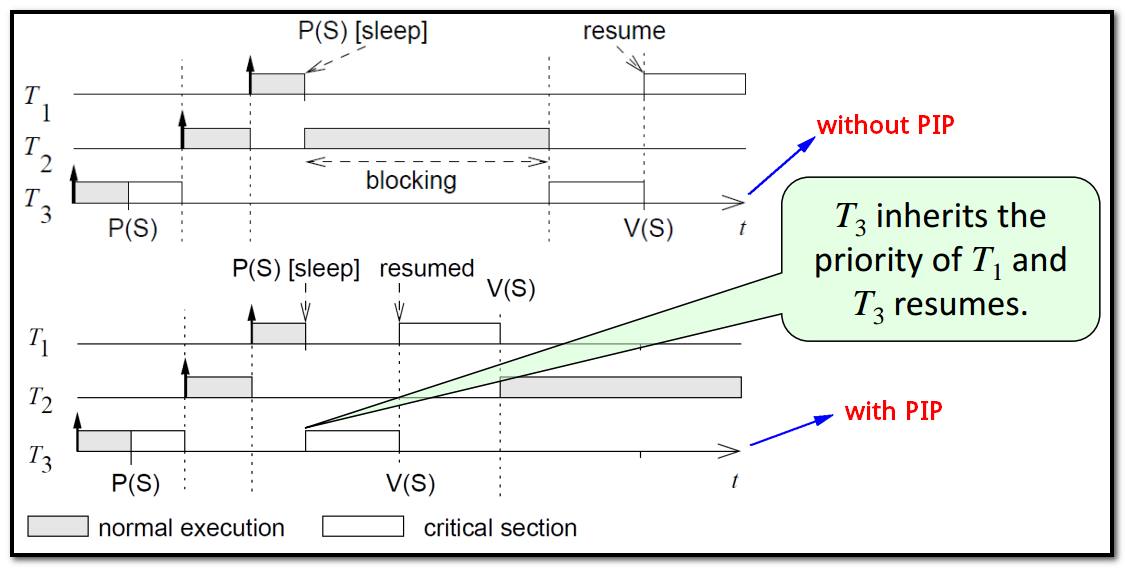• Direct Blocking: higher-priority task $T_1$ tries to acquire a resource held by a lower priority task $T_3$.
• Push-through Blocking: medium-priority task $T_2$ is blocked by a lower-priority task $T_3$ that has inherited a higher priority from a task ($T_1$) it directly blocks.
• Tasks have nominal（挂名优先级） and active（活跃优先级） priorities
• Nominal priority: assigned by the scheduling algorithm, e.g. $T_1$’s priority.

static priorities or dynamic priorities 取决于 nominal priorities 的情况

• Active priority: assigned by the priority inheritance protocol dynamically to avoid priority inversion, e.g. $T_3$’s priority inherited from $T_1$.
• PIP Algorithm
• Tasks are scheduled according to their active priorities. Tasks with the same priorities are scheduled FCFS.
• Task $T_i$’s nominal priority $P_i$, task $T_k$’s nominal priority $P_k$.
• When a task $T_i$ tries to enter a critical section and the resource is blocked by a lower priority task $T_k$, the task $T_i$ is blocked. Otherwise it enters the critical section.
• When a task $T_i$ is blocked, it transmits its active priority to the task $T_k$ that holds the semaphore.
• $T_k$ resumes and executes the rest of its critical section with a priority $p_k=max(p_i)$, it inherits the priority of the highest priority of the tasks blocked by it.
• When $T_k$ exits a critical section, it unlocks the semaphore and the highest priority task blocked on that semaphore is awakened.
• If no other tasks are blocked by $T_k$, then $p_k$ is set to its nominal priority $P_k$.
• Otherwise it is set to the highest priority of the left tasks blocked by $T_k$.
• Thus, medium priority tasks which do no share resources with $T_k$ cannot preempt $T_k$ and cannot prolong the blocking（延长阻塞） of the higher priority tasks
• Nested Critical Sections（一个临界区内嵌套了另一个临界区）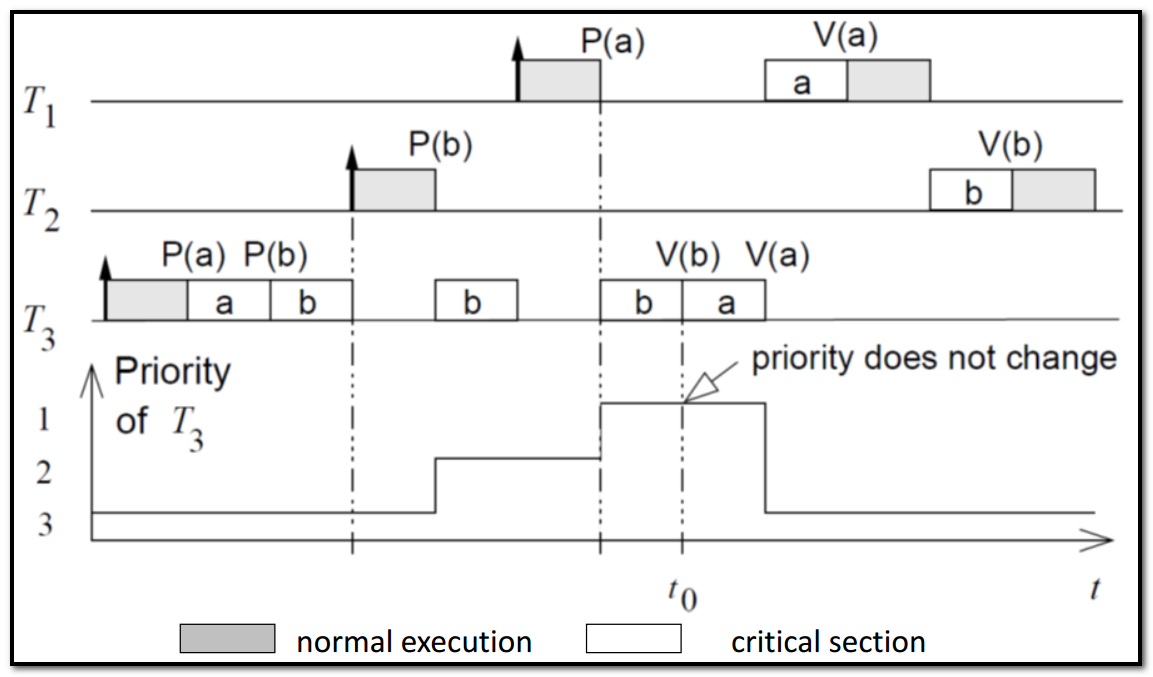PIP for Nested Critical Sections, $S_b$ nested in $S_a$.
• Transitiveness of Priority Inheritance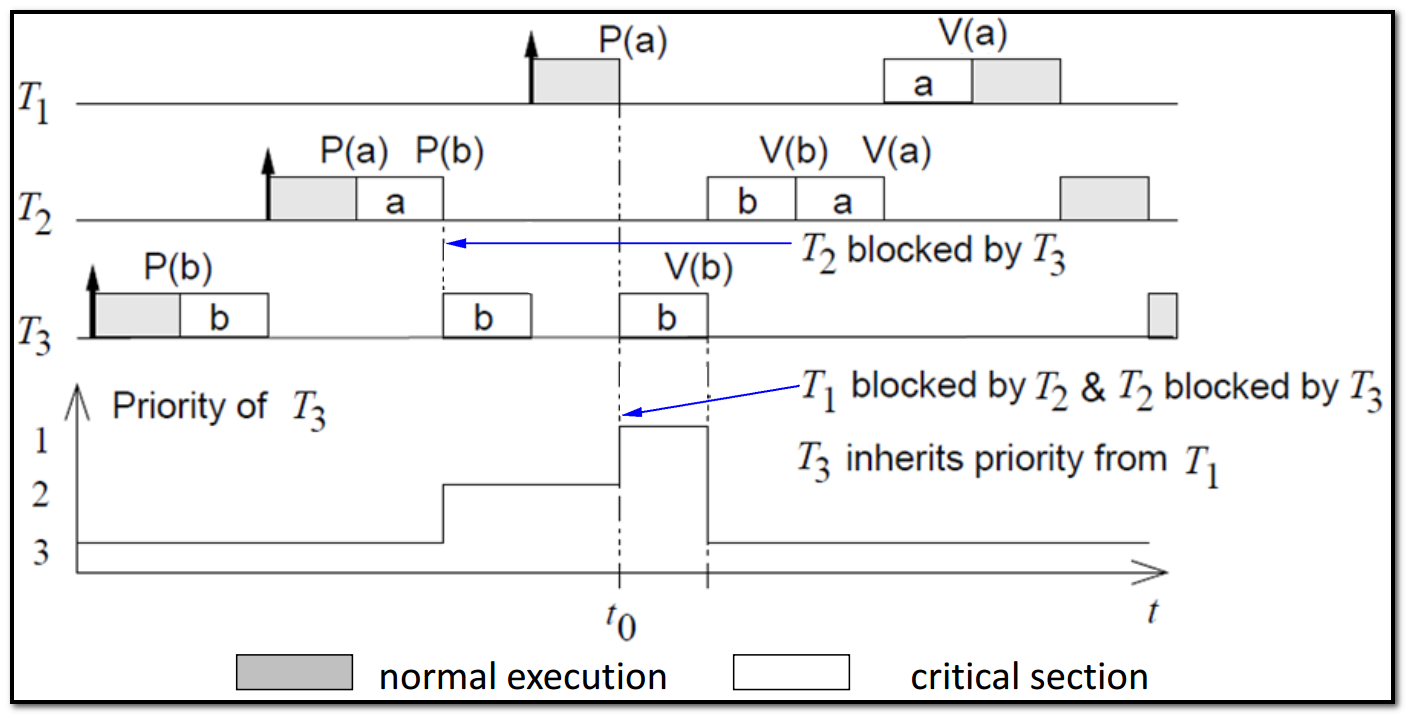If $T_1$ is blocked by $T_2$ and $T_2$ is blocked by $T_3$, then $T_3$ inherits the priority of $T_1$ via $T_2$
• Pros and Cons
• Under the priority inheritance protocol, a job $J$ can be blocked for at most the duration of $min(n, m)$ critical sections, where $n$ is the number of lower-priority jobs that could block $J$ and $m$ is the number of distinct semaphores that can be used to block $J$.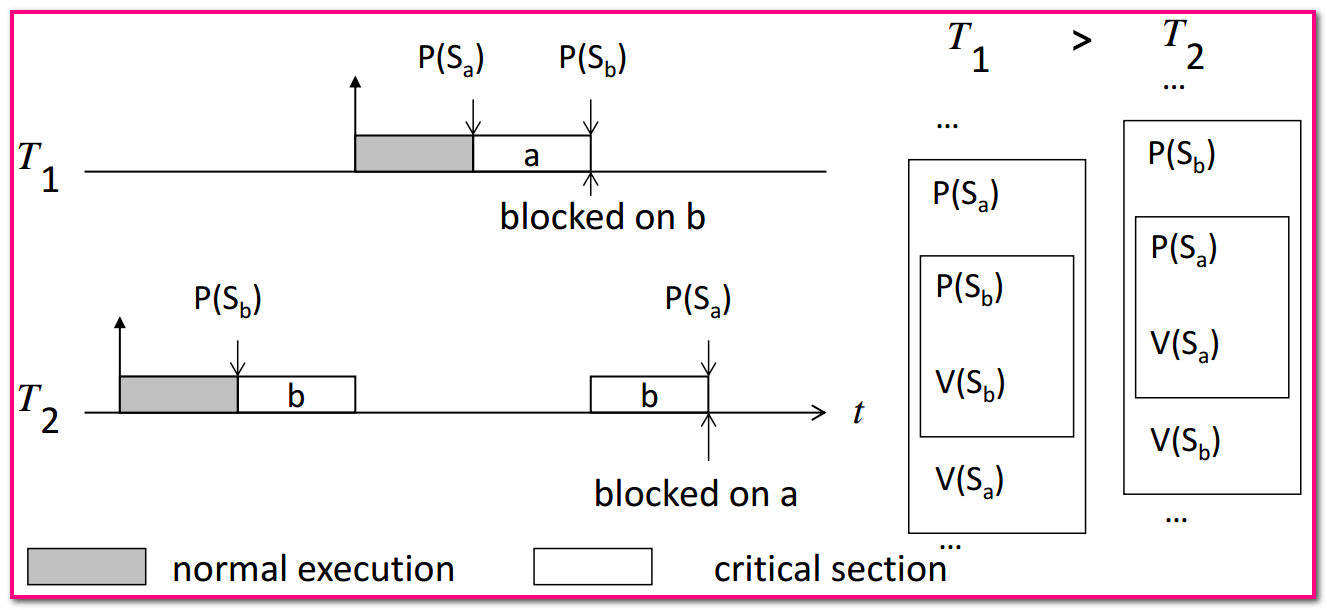• Chained Blocking: $J$ can get blocked on $n$ critical sections held by $n$ distinct lower priority jobs.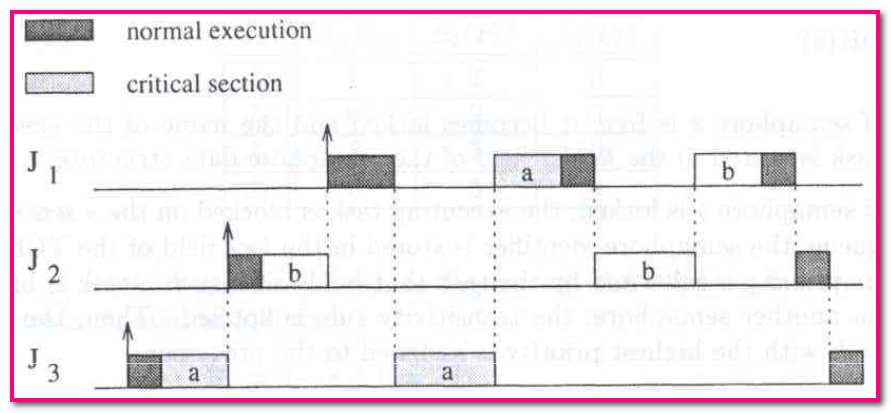$J_1$ is blocked for the duration of two critical sections, once to wait $J_3$ to release $S_a$ and then to wait $J_2$ to release $S_b$.

### Priority Ceiling Protocol (PCP)

• PIP $\rightarrow$ PCP: extension of Priority Inheritance Protocol to handle chained blocking and deadlocks
• avoids multiple blocking
• guarantees that, once a task has entered a critical section, it cannot be blocked by lower priority tasks until its completion
• PCP Algorithm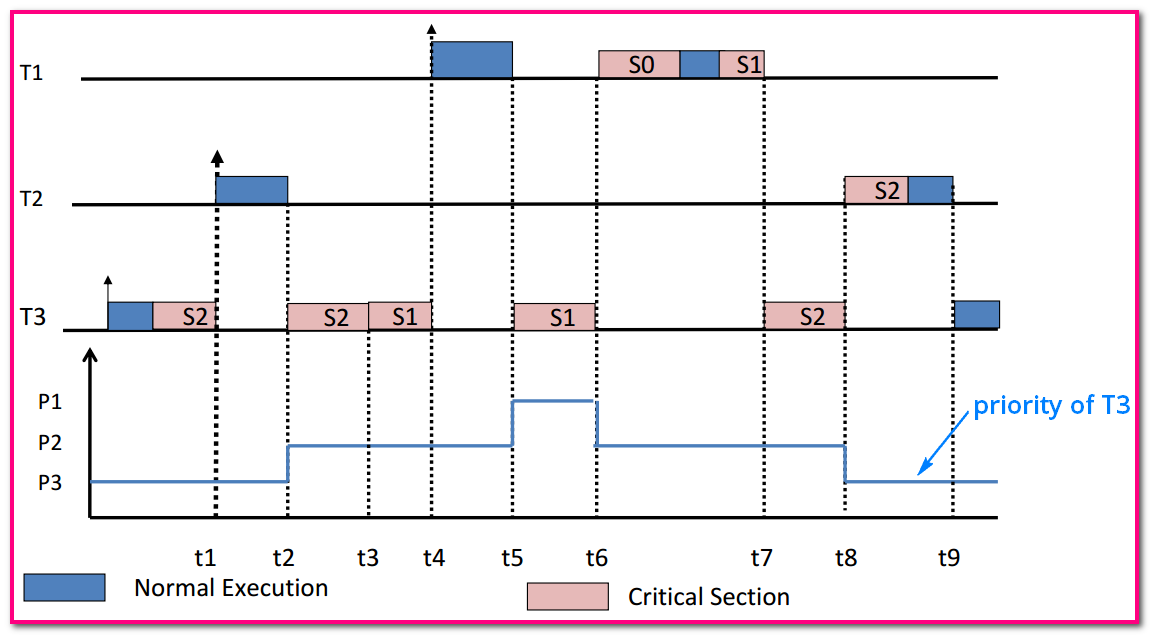Task $T_1$, $T_2$, $T_3$ and their corresponding priorities $P_1$ > $P_2$ >$P_3$
• Each semaphore $S_k$ is assigned a priority ceiling $C(S_k)$. It is the priority of the highest priority task that can lock $S_k$. This is a static value.

$C(S_0) = P_1$　　$C(S_1) = P_1$　　$C(S_2) = P_2$

• Suppose $T_i$ is currently running and it wants to lock the semaphore $S_k$.
• $T_i$ is allowed to lock $S_k$ only if the priority of $T_i$ is strictly higher than the priority ceiling $C(S_k)$ of the semaphore $S_k$ where: $S_k$ is the semaphore with the highest priority ceiling among all the semaphores which are currently locked by tasks other than $T_i$

检测到自身优先级比所有被占有的资源的 ceiling 值都高的时候才可以获取到请求的资源；而且，当存在资源被其它任务占有的时候，才需要进行检测操作

• Otherwise, $T_i$ is said to be blocked by the semaphore $S_k$ (and the tasks currently holding $S_k$). When $T_i$ gets blocked by $S_k$ then the priority of $T_i$ is transmitted to the tasks $T$ that currently holds $S_k$.

At time t2: $T_2$ can not lock $S_2$. Currently $T_3$ is holding $S_2$ and $C(S_2) = P_2$ and the current priority of $T_2$ is also $P_2$.
At time t5: $T_1$ can not lock $S_0$. Currently $T_3$ is holding $S_2$ and $S_1$ and $C(S_1) = T_1$ and the current priority of $T_1$ is also $P_1$. The (inherited) priority of $T_3$ is now $P_1$.

• When $T_i$ leaves a critical section guarded by $S_k$ then it unlocks $S_k$ and the highest priority task, if any, which is blocked by $S_k$ is awakened. The priority of $T_i$ is set to the highest priority of the task that is blocked by other semaphores that $T_i$ is still holding. If none, the priority of $T_i$ is set to be its nominal one.

At time t6: $T_3$ unlocks $S_1$. It awakens $T_1$. But $T_3$’s (inherited) priority is now only $P_2$ while $P_1 > C(S_2) = P_2$. So $T_1$ preempts $T_3$ and runs to completion.
At time t7: $T_3$ resumes execution with priority $P_2$.
At time t8: $T_3$ unlocks $S_2$ and goes back to its nominal priority $P_3$. So $T_2$ preempts $T_1$ and runs to completion.

• Results
+ A task is not allowed to enter a critical section if there are already locked semaphores which could block it eventually.
+ Hence, once a task enters a critical section, it can not be blocked by lower priority tasks（不会被较当前任务低优先级的任务阻塞）till its completion.
• PCP: Properties
+ A given task $T$ is delayed at most once by a lower priority task. The delay is a function of the time taken to execute the critical section
+ Deadlock free (only changing priorities)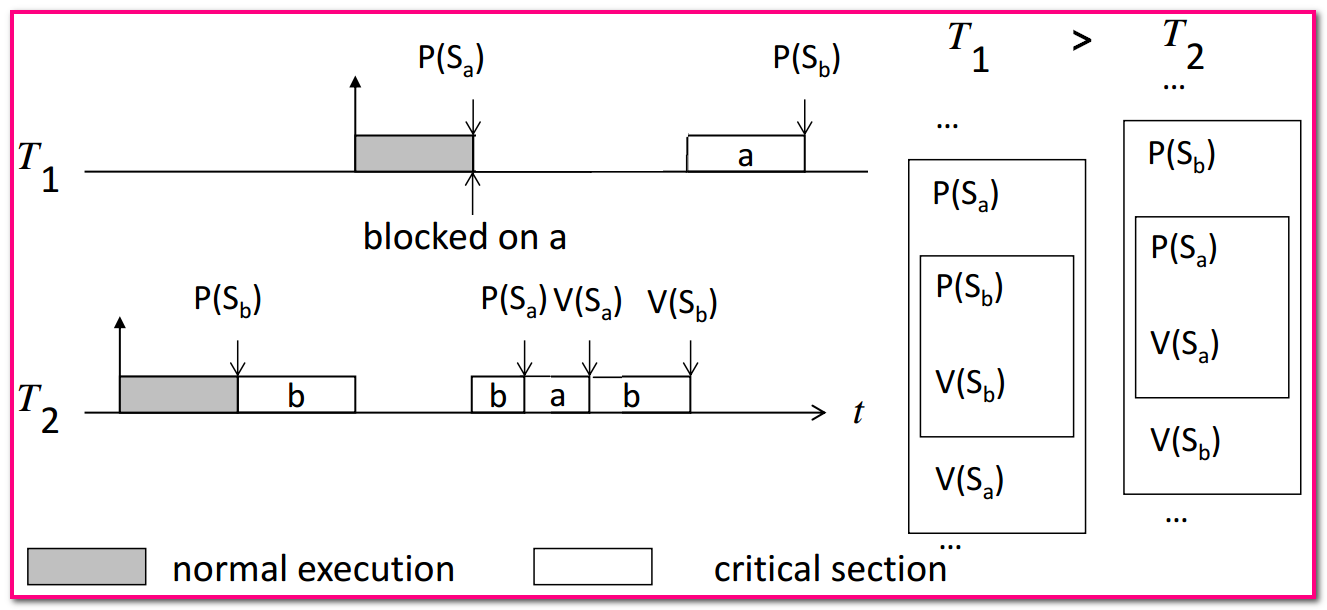Deadlock free using PCP with $ceil(S_1)=P_1$, $ceil(S_2)=P_1$

### Stack Resource Policy (SRP)

• PCP Extending: Stack Resource Policy (SRP)
• SRP supports dynamic priority scheduling
• PCP blocks a task at the time it makes the resource request, while SRP blocks task at the time it attempts to preempt.
• SRP Algorithm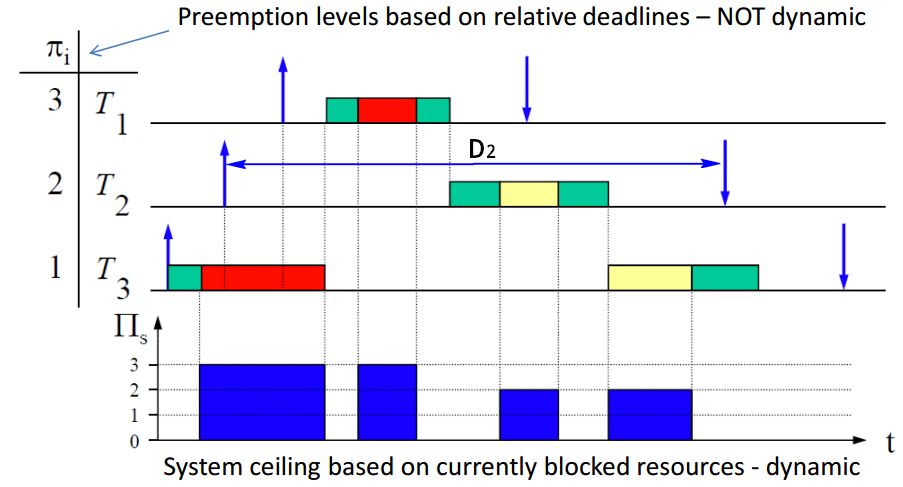• Preemption Level: $\pi _ i \propto \frac{1}{D_i}$, tasks $T_i$ with larger deadlines $D_i$ have lower preemption level (Intuition: they can be easily preempted), is a static value.
• Resource ceiling: of a resource is the highest preemption level from amongst of all tasks that may access that resource. Note: (i) this is associated with each resource (ii) this is static.

Resource ceiling for the red resource is 3. For the yellow resource, it is 2.

• System ceiling: is the highest resource ceiling level from amongst of resources that are currently blocked (当前阻塞任务的所有资源的 Resource ceiling 的最大值). Note: (i) this is not associated with each resource but with the system (ii) this is a dynamic parameter that can change every time a resource is accessed or released.

Based on this, we can see how the system ceiling $\prod _s$ varies dynamically.

• it has the highest priority;
• and its preemption level is higher than the system ceiling. $\pi _ i > \prod _s$

Task $T_3$ is not preempted by $T_2$ even though it does not share the red resource ($\pi _2 < \prod _s$).

• SRP reduces preemptions compared to PCP.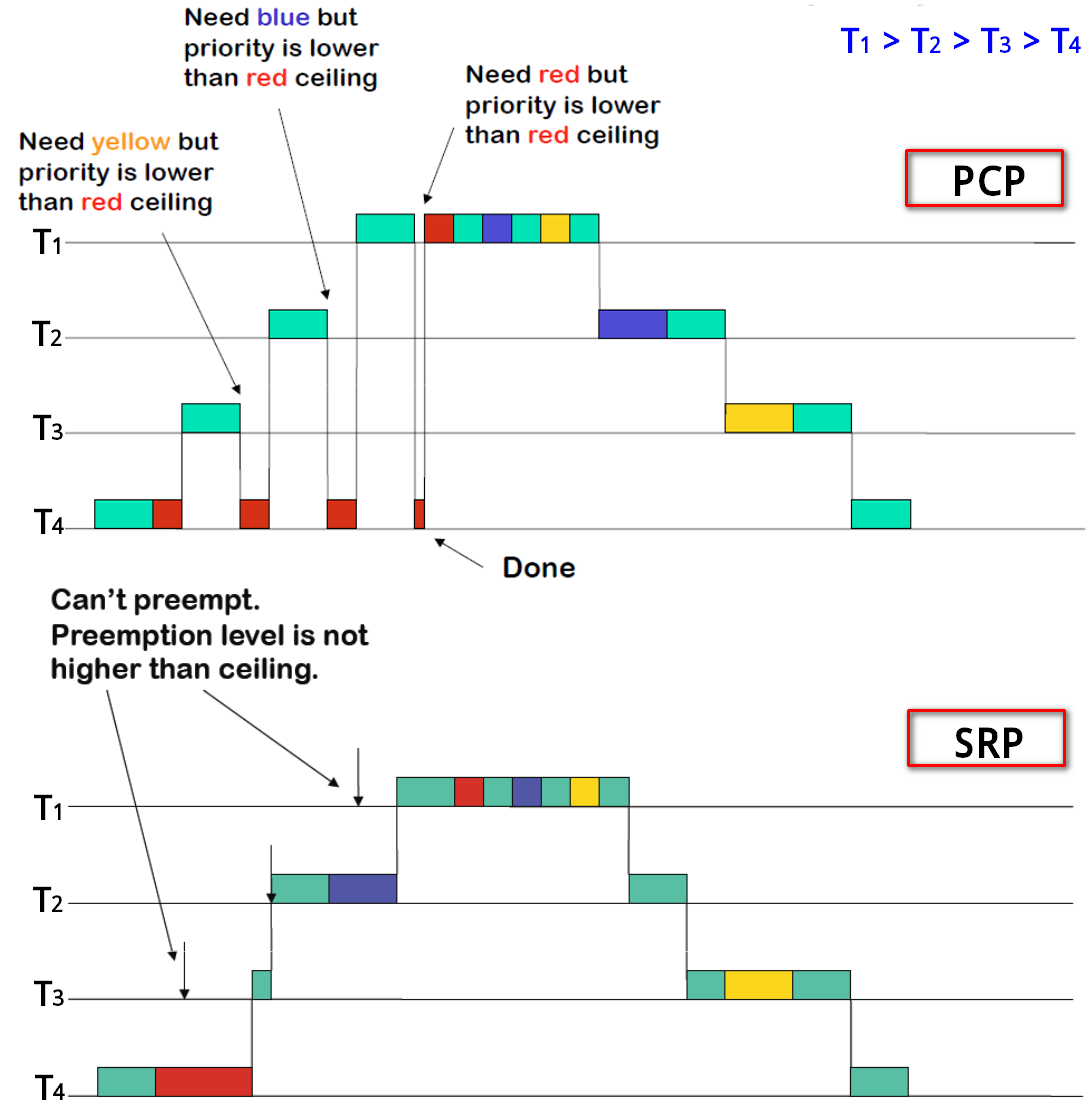• 优先级<SystemCeiling 的进程最终会被阻塞，或因为请求访问临界区，或因为被高优先级抢占，考虑到这一点，SRP 相比于 PCP 能够明显减少 Context Switch.

A task cannot be blocked by tasks with lower premption levels (these tasks can resume only when the task completes). Hence, if there are tasks on the same preemption level, they can never occupy stack space on the same time. Higher the number of tasks on the same preemption level, larger the stack space saving!

• When a task needs a resource that is not available, it is blocked at the time it attempts to preempt, rather than later.
• To prevent multiple priority inversions, a task is not allowed to start until the resource currently available are sufficient to meet the maximum requirement of every task that could preempt it.
• Slides from Introduction to embedded systems, Kai Huang, School of Data and Computer Science, Sun Yat-sen University, China. All rights reserved.
• E. A. Lee and S. A. Seshia, Introduction to Embedded Systems - A Cyber-Physical Systems Approach, LeeSeshia.org, 2011.